# Sparse flux balance analysis test for a minimial stoichiometrically balanced cycle involving ATP hydrolysis

## INTRODUCTION

We consider a biochemical network of m molecular species and n biochemical reactions. The biochemical network is mathematically represented by a stoichiometric matrix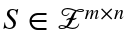. In standard notation, flux balance analysis (FBA) is the linear optimisation problemwhere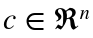is a parameter vector that linearly combines one or more reaction fluxes to form what is termed the objective function, and where a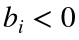, or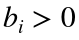, represents some fixed output, or input, of the ith molecular species. A typical application of flux balance analysis is to predict an optimal non-equilibrium steady-state flux vector that optimises a linear objective function, such biomass production rate, subject to bounds on certain reaction rates.
In this tutorial, we demonstrate how to predict the minimal number of active reactions that are still consistent with an optimal objective derived from the result of a standard flux balance analysis problem. In each case, the corresponding problem is a cardinality minimisation problem that we term sparse flux balance analysiswhere the last constraint is optional and represents the requirement to satisfy an optimal objective value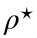derived from any solution to a flux balance analysis (FBA) problem. This approach is used to check for minimal sets of reactions that either should be active, or should not be active in a flux balance model that is representative of a biochemical network.
In particular, we use sparse flux balance analysis test for a minimal stoichiometrically balanced cycle involving ATP hydrolysis, which should never appear in any flux balance analysis model where constraints arising from ATP demands are being implemented, since a stoichiometrically balanced cycle involving ATP hydrolysis might create artefactual energy metabolism predictions. In order to mimic the requirement for energy, for maintenance of cellular integrity, many flux balance models contain a cytoplasmic adenosine triphosphate (atp[c]) hydrolysis reaction where the products are adenosine diphosphate (adp[c]) and orthophosphate (pi[c]). In Recon 3D, the full corresponding reaction formula is
h2o[c] + atp[c] -> h[c] + adp[c] + pi[c] (1)
In a flux balance model, a maintenance requirement for synthesis of adenosine triphosphate can be represented with a lower bound on reaction (1) or inclusion of reaction (1) within a composite biomass reaction, when cellular growth is being modelled . In order for either of these approaches to result in a constraint on energy metabolism within the model, no stoichiometrically balanced set of internal reactions that include reaction (1) should admit isolated hydrolysis of ATP, given the reaction bounds supplied with the model. If such a set exists, sparse flux balance analysis can be used to find one such minimal cardinality set [4, 5].

## TIMING

A minimal solution to sparse flux balance analysis problem can be obtained in < 10 seconds. The time consuming part is comparing the predictions with the biochemical literature to assess whether the predictions are consistent with biochemical network function or not, as such, the process of refining a model to increase its biochemical fidelity can take days or weeks.

## EQUIPMENT SETUP

Make sure to initialise the COBRA Toolbox.
initCobraToolbox(false) % false, as we don't want to update

## Setting the numerical tolerance

Implementation of sparse flux balance analysis with any numerical optimisation solver requires a tolerance to be set that distinguished between zero and non-zero flux, based on the numerical tolerance of the currently installed optimisation solver. Typically 1e-6 will suffice, except for multiscale models.
feasTol = getCobraSolverParams('LP', 'feasTol');

We are going to focus here on testing the biochemical fidelity of Recon3.0model . As an example, Recon 1.0 may also be used .
global CBTDIR
filename = 'Recon1.0model.mat'; % amend fileName for Recon 3
model = readCbModel([CBTDIR filesep 'test' filesep 'models' filesep filename]);
model.csense(1:size(model.S,1),1) = 'E';

## Testing for production of ATP with all external reactions blocked, but all internal reaction bounds unchanged

There are two options: A: sparse flux balance analysis using zero norm minimisation, and B: one norm minimisation.

## A: Sparse flux balance analysis test for production of ATP with all external reactions blocked, but all internal reaction bounds unchanged

Detect the ATP maintenance reaction and if there is none already, add one.
atpMaintenanceBool=strcmp(model.rxns,'DM_atp_c_') | strcmp(model.rxns,'DM_atp(c)') | strcmp(model.rxns,'ATPM');
if ~any(atpMaintenanceBool)
fprintf('Could not find ATP maintenance reaction, adding one.')
if ~strcmp(filename,'HMR2.0')
model = addReaction(model, 'ATPMnew', 'h2o[c] + atp[c] -> h[c] + adp[c] + pi[c]');
else
model = addReaction(model, 'ATPMnew', 'm02040c + m01371c -> m02039c + m01285c + m02751c');
end
atpMaintenanceBool=strcmp(model.rxns,'ATPMnew');
fprintf('%s %s\n',model.rxns{atpMaintenanceBool},' is the ATP maintenance reaction')
else
fprintf('%s %s\n',model.rxns{atpMaintenanceBool},' is the ATP maintenance reaction:')
end
Display the size of the model
[nMet,nRxn] = size(model.S);
fprintf('%6s\t%6s\n','#mets','#rxns'); fprintf('%6u\t%6u\t%s%s\n',nMet,nRxn,' totals in ', filename)
Display the constraints
minInf = -1000;
maxInf = 1000;
printConstraints(model, minInf, maxInf);
Identify the exchange reactions(s) heuristically
if ~isfield(model,'SIntRxnBool')
model = findSExRxnInd(model,size(model.S,1),1);
end
Maximise the atp maintenance reaction
model.c(:) = 0;
model.c(atpMaintenanceBool) = 1;
osenseStr = 'max';
Choose to minimize the zero norm of the optimal flux vector
minNorm = 'zero';
Allow thermodynamically infeasible fluxes
allowLoops = 1;
Select the approximate step functions when minimising the zero norm of the flux vector
% zeroNormApprox='cappedL1';% : Capped-L1 norm
% zeroNormApprox='exp';%Exponential function
% zeroNormApprox='log';%Logarithmic function
% zeroNormApprox='SCAD';%Smoothly clipped absolute deviation function
% zeroNormApprox='lp-';%L_p norm with p<0
% zeroNormApprox='lp+';%L_p norm with 0<p<1
zeroNormApprox='all';% test all approximations avialable and return the best one
Close all external reactions
model.lb(~model.SIntRxnBool) = 0;
model.ub(~model.SIntRxnBool) = 0;
Run sparse flux balance analysis on the model with all exchanges closed
tic
sparseFBAsolutionBounded = optimizeCbModel(model, osenseStr, minNorm, allowLoops, zeroNormApprox);
toc
Check to see if there is a non-zero flux through the ATP maintenance reaction
fprintf('%g%s\n',sparseFBAsolutionBounded.v(atpMaintenanceBool),' flux through the ATP maintenance reaction')
Display the sparse flux solution, but only the non-zero fluxes, above a specified cutoff.
cutoff=0.1;
for n=1:nRxn
if abs(sparseFBAsolutionBounded.v(n))>cutoff
formula=printRxnFormula(model, model.rxns{n}, 0);
fprintf('%10g%15s\t%-60s\n',sparseFBAsolutionBounded.v(n),model.rxns{n}, formula{1});
end
end

## ANTICIPATED RESULTS

When all external reactions are blocked (bounds are set to zero), then the only net flux admissible is within a stoichiometrically balanced cycle, if and only if, the bounds on each reaction in the stoichiometrically balanced cycle simultaneously admit net flux in one direction around the cycle. Net flux around a stoichiometrically balanced cycle is thermodynamically infeasible , but steady state mass balance constraints do not enforce thermodynamic constraints. In lieu of such constraints, the bounds on reactions can be set based on the biochemical literature to eliminate net flux around a stoichiometrically balanced cycle. In Recon3.0, with all external reactions blocked, maximising reaction (1) while minimising the cardinality of all internal reactions, using sparse flux balance analysis was used to find one such minimal cycle. The optimal solution involves reaction (1) in a set of nine stoichiometrically balanced reactions, with bounds that admit an arbitrary amount of isolated ATP hydrolysis. Recon3.0model contains no set of reactions that admit an arbitrary amount of isolated ATP hydrolysis.

## TROUBLESHOOTING

By further constraining the bounds to convert one reversible reaction in each such stoichiometrically balanced cycle to an irreversible reaction, isolated ATP hydrolysis can be eliminated, e.g., though there are important exceptions, a reactions hydrolyses ATP does not generally operate in a reverse direction at biochemically realistic metabolite concentrations.

## B: One norm minimisation test for production of ATP with all external reactions blocked, but all internal reaction bounds unchanged

Run flux balance analysis on the same model and minimise the total sum of all reaction rates (minimum one norm)
minNorm = 'one';
oneNormFBASolutionBounded = optimizeCbModel(model, osenseStr, minNorm, allowLoops, zeroNormApprox);
Display the one norm flux balance analysis solution, but only the non-zero fluxes, above a specified cutoff.
for n=1:nRxn
if abs(oneNormFBASolutionBounded.v(n))>cutoff
formula = printRxnFormula(model, model.rxns{n}, 0);
fprintf('%10g%15s\t%-60s\n',oneNormFBASolutionBounded.v(n),model.rxns{n}, formula{1});
end
end

## ANTICIPATED RESULTS

Depending on the model, minimising the one norm may give as good an approximation of a minimal stoichiometrically balanced cycle as minimising the zero norm, but experience suggests this is less likely for large cycles or large models.

## Testing for production of ATP with all external reactions blocked and all internal reactions reversible

There are two options: A: sparse flux balance analysis using zero norm minimisation, and B: one norm minimisation.

## A: Sparse flux balance analysis test for production of ATP with all external reactions blocked and all internal reactions reversible

Fully open all internal reactions
model.lb(model.SIntRxnBool) = -1000;
model.ub(model.SIntRxnBool) = 1000;
Run sparse flux balance analysis on the model with all exchanges closed and all internal reactions reversible
sparseFBAsolutionUnBounded = optimizeCbModel(model, osenseStr, minNorm, allowLoops, zeroNormApprox);
Check to see if there is a non-zero flux through the ATP maintenance reaction
fprintf('%g%s\n',sparseFBAsolutionUnBounded.v(atpMaintenanceBool),' flux through the ATP maintenance reaction');
Display the sparse flux solution, but only the non-zero fluxes, above a specified cutoff.
cutoff=0.1;
for n=1:nRxn
if abs(sparseFBAsolutionUnBounded.v(n))>cutoff
formula=printRxnFormula(model, model.rxns{n}, 0);
fprintf('%10g%15s\t%-60s\n',sparseFBAsolutionUnBounded.v(n),model.rxns{n}, formula{1});
end
end

## ANTICIPATED RESULTS

When all reactions are reversible, in a genome-scale model, it should be anticipated to find a stoichiometrically balanced cycle of reactions that admit an arbitrary amount of isolated ATP hydrolysis. It is important nevertheless to realise that these cycles are latent in the network and could become active with inadvertent relaxation of model bounds.

## B: One norm minimisation test for production of ATP with all external reactions blocked and all internal reactions reversible

Run flux balance analysis on the samemodel and minimise the sum total of all reaction rates (minimium one norm)
minNorm = 'one';
oneNormFBASolutionUnBounded = optimizeCbModel(model, osenseStr, minNorm, allowLoops, zeroNormApprox);
Display the one norm flux balance analysis solution, but only the non-zero fluxes, above a specified cutoff.
for n=1:nRxn
if abs(oneNormFBASolutionUnBounded.v(n))>cutoff
formula = printRxnFormula(model, model.rxns{n}, 0);
fprintf('%10g%15s\t%-60s\n',oneNormFBASolutionUnBounded.v(n),model.rxns{n}, formula{1});
end
end

## ANTICIPATED RESULTS

When all reactions are reversible, in a genome-scale model, it should be anticipated to find a stoichiometrically balanced cycle of reactions that admit an arbitrary amount of isolated ATP hydrolysis. It is important nevertheless to realise that these cycles are latent in the network and could become active with inadvertent relaxation of model bounds.

## REFERENCES

 Feist, Adam M, and Bernhard O Palsson. “The Biomass Objective Function.” Current Opinion in Microbiology, Ecology and industrial microbiology • Special section: Systems biology, 13, no. 3 (June 2010): 344–49. doi:10.1016/j.mib.2010.03.003.
 Fleming, R. M. T., C. M. Maes, M. A. Saunders, Y. Ye, and B. Ø. Palsson. “A Variational Principle for Computing Nonequilibrium Fluxes and Potentials in Genome-Scale Biochemical Networks.” Journal of Theoretical Biology 292 (January 7, 2012): 71–77. doi:10.1016/j.jtbi.2011.09.029.
 Brunk, Elizabeth, Swagatika Sahoo, Daniel C Zielinski, Ali Altunkaya, Maike Aurich, Nathan Mih, German Andres Preciat Gonzalez, et al. “Recon 3D: A Resource Enabling a Three-Dimensional View of Gene Variation in Human Metabolism.” (Submitted) 1 (2017).
 Fleming, R.M.T., et al., Cardinality optimisation in constraint-based modelling: illustration with Recon 3D (submitted), 2017.
 Le Thi, H.A., Pham Dinh, T., Le, H.M., and Vo, X.T. (2015). DC approximation approaches for sparse optimization. European Journal of Operational Research 244, 26–46.
 Duarte, N. C., S. A. Becker, N. Jamshidi, I. Thiele, M. L. Mo, T. D. Vo, R. Srivas, and B. Ø. Palsson. “Global Reconstruction of the Human Metabolic Network Based on Genomic and Bibliomic Data.” Proceedings of the National Academy of Sciences of the United States of America 104, no. 6 (2007): 1777–82. doi:10.1073/pnas.0610772104.